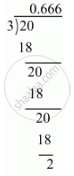# Express the Following Rational Number as Decimal: 2/3 - Mathematics

Express the following rational number as decimal:

2/3

#### Solution

Given rational number is 2/3

Now we have to express this rational number into decimal form. So we will use long division methodTherefore 2/3=0.6666

rArr2/3=0.bar6

Concept: Introduction of Real Number
Is there an error in this question or solution?

#### APPEARS IN

RD Sharma Mathematics for Class 9
Chapter 1 Number Systems
Exercise 1.2 | Q 2.1 | Page 13
Share